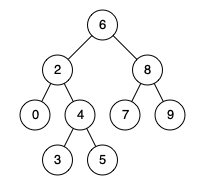# Leetcode 235 - LCA of BST

Note:

• Much like 236, use recursion - If root.val is between p.val and q.val, it means we found the result.
• Base: we found the result - root.val is in [p.val, q.val] (Assume p.val is smaller than q.val)
• If root.val is bigger than both of them, then we need to search in smaller values, whcih means we need to get into left subtree.
• Likewise, do right subtree.

Given a binary search tree (BST), find the lowest common ancestor (LCA) of two given nodes in the BST.

According to the definition of LCA on Wikipedia: “The lowest common ancestor is defined between two nodes p and q as the lowest node in T that has both p and q as descendants (where we allow a node to be a descendant of itself).”

Example